# SAT Math Multiple Choice Question 53: Answer and Explanation

### Test Information

Question: 53

3.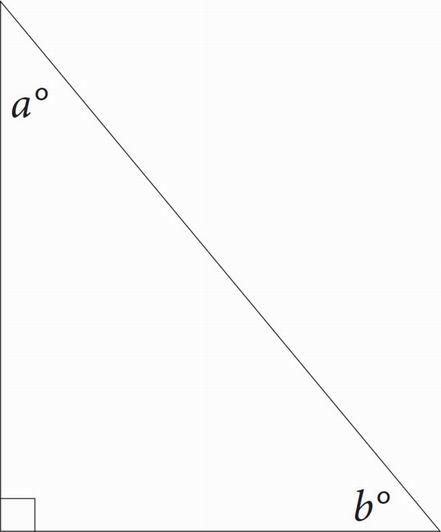In the figure above, sin a = x. What is the value of cos b?

• A. x
• B.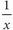• C. |1 - x|
• D.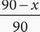A You can plug in when you're dealing with a geometry problem with unknowns. When you're Plugging In for a right triangle, use one of the special right triangles to make your life easier. Use a 3-4-5 right triangle. Make the side opposite a 3, the side adjacent to a 4, and the hypotenuse 5. Because sine is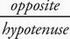, sin a =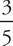, so x =. Cosine is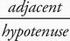, so cosb =. This is your target; circle it. Make x =in each answer choice and look for the answer which equals. Only (A) works.